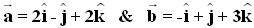# CBSE Class 12th Mathematics Practice Paper Set-I

50 Questions | Total Attempts: 483SettingsThis practice paper is completely based on the CBSE class 12thMathematics question paper 2013 in MCQ form to build you concept in class 12thMathematics This practice paper can also be helpful for JEE For example: Q. Write unit vector in the direction of the sum of vectors: The above given question could be asked in JEE in the following way: Q. Write unit vector in the direction of the sum of vectors: Learn and judge yourself with jagranjosh. Com practice question paper that how question are being framed in CBSE class 12th board exam as well as in JEE

• 1.
Find the direction cosines of the following equation of line:
• A.
• B.
• C.
• D.
• 2.
Find the Cartesian equation of the line which passes through the point (-2, 4, -5) and is parallel to the line:
• A.
• B.
• C.
• D.
• 3.
Write unit vector in the direction of the sum of vectors:
• A.
• B.
• C.
• D.
• 4.
• A.

1

• B.

-1

• C.

2

• D.

0

• 5.
• A.

2

• B.

3

• C.

4

• D.

2

• 6.
The amount of pollution content added in air in a city due to x-diesel vehicles is given by  Find the marginal increase in pollution content when 3 diesel vehicles are added.
• A.

30.522

• B.

32.055

• C.

31.255

• D.

30.255

• 7.
• A.
• B.
• C.
• D.
• 8.
• A.
• B.
• C.

14

• D.

2

• 9.
Find the value of a?
• A.

1

• B.

4

• C.

2

• D.

-1

• 10.
Find the value of b+c
• A.

7

• B.

2

• C.

5

• D.

6

• 11.
Find the value of c + d
• A.

1

• B.

2

• C.

5

• D.

7

• 12.
• A.
• B.

12

• C.
• D.
• 13.
• A.
• B.
• C.
• D.
• 14.
• A.
• B.
• C.
• D.
• 15.
A speaks truth in 75% of the cases, while B in 90% of the cases. In what percent of cases are they likely to contradict each other in stating the same fact?
• A.

20 %

• B.

10 %

• C.

30 %

• D.

40 %

• 16.
A line intersects the plane x - y + z - 5 = 0. Find the angle between the line & the plane.
• A.
• B.
• C.
• D.
• 17.
Find the coordinates of the point, where the line intersects the plane x - y + z - 5 = 0.
• A.

(2, 1, 2)

• B.

(-2, 1, 2)

• C.

(2, -1, 2)

• D.

(2, -1, -2)

• 18.
Find the vector equation of the plane which contains the line of intersection of the planes  & which is perpendicular to the plane
• A.
• B.
• C.
• D.
• 19.
Using vectors, find the area of the triangle ABC with vertices A (1, 2, 3), B (2, -1, 4) and C (4, 5, -1).
• A.
• B.
• C.
• D.
• 20.
• A.
• B.
• C.
• D.
• 21.
• A.
• B.
• C.
• D.
• 22.
• A.
• B.
• C.
• D.
• 23.
• A.
• B.
• C.
• D.
• 24.
• A.

Cos t

• B.

Cot t

• C.

Sin t

• D.

Tan t

• 25.
• A.
• B.
• C.
• D.
Related TopicsBack to top